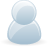# Support Forum

or3373 Views - Created 12/06/2016

12/06/2016

Posted by:
sandeeptara@gmail.comi am trying to read pt100 RTDs using channel 1 and channel 2 . I have tested everything using excel and external voltage outside of Raspberry Pi and expended Pi I am getting perfect temperature measurements using Wheatstone bridge and callendar-van dusen equation when I measure using my digital multi meter. I believe that when connecting to expander Pi I need to join both grounds , (I am using 2,5Volts constant power & of course removed jumper) and output to pin#1 & #2. I tried that but got readings close to Vref that was kept at default 4.096V initially in the program, it read 4.095V when I ran example program. Can someone share application notes on it? I tried to put Vref =2.5 too but reads similarly close to Vref 2.499 When I ran program. By multimeter it reads 0 .538V. I have not connected anything to pin 15 Vref

12/06/2016

Posted by:
andrewLocation:
United Kingdom

I think the problem could be that the ADC is configured to read in single-ended mode instead of differential mode so it is reading the voltage between each input and ground which on a Wheatstone bridge would read 2.5V as the voltage will be going directly from V+ through one of the resistors to the ADC input and into ground. To measure the difference across the Wheatstone bridge the ADC will need to be set to differential mode so it measures the difference between channels 1 and 2.

At the moment none of our Expander Pi libraries supports differential mode on the Expander Pi ADC but if you let me know which language you are using I will update that library so you can use it in differential mode and hopefully read the RTD correctly.

12/06/2016

Posted by:
andrewLocation:
United Kingdom

After thinking a bit more about your problem I think it could be that you need to connect the vref pin to your 2.5V constant power source. If you have removed the jumper shown in the red circle below then the ADC reference will not be connected to anything so it will be measuring between ground and 0V so any input would return as the maximum value. If you connect your 2.5V to the vref pin then that will be used as the maximum reference and the Expander Pi should return the correct values.14/06/2016

Posted by:
sandeeptara@gmail.comThanks - to connect 2.5V was important. It is working. Only issue is -Chanel 1 and 2 can't be chosen. I had to use 1 and 3 . If I use Chanel 1 and 2 readings get messed up.

16/06/2016

Posted by:
sandeeptara@gmail.comI am able to read voltage using expender pi, but python code for resistance and Callendar–Van Dusen equation which works great in excel do not work in python .Both equations work in excel T=((-(H3*H6)+SQRT(H6*H6*H3*H3-4*(H6*H4)*(H6-(D10))))/(2*H4*H6))*9/5+32or T=((-H3)+SQRT(H3*H3-4*H4*(1- D10/100)))/(2*H4)*9/5+32where H3=a=3.9083*10^-3 H4=b=-5.775*10^-7 , H5=c=-5.775*10^-7, R0=H6=100, D10=Rrtd. I am using 270Ohms resistnce at divider , Rrtd =270/((D2/D3)-1) D2= VinD3=Voutany suggestions?

16/06/2016

Posted by:
andrewLocation:
United Kingdom

I have tried to recreate your first equation in excel and python and managed to get a script working that gives the same output.#!/usr/bin/pythonimport mathD2 = 2.0D3 = 1.0DividerRes = 270t = 0.0H3 = 0.0039083H4 = -0.0000005775H5 = 0.0000005775H6 = 100D10 = DividerRes/((D2/D3)-1)a = H6*H6*H3*H3-4*(H6*H4)*(H6-(D10))b = math.sqrt(a)t = ((-(H3*H6)+b)/(2*H4*H6))*9/5+32print aprint bprint tThe above code gives a result of 873.011583507 in python and 873.0116 in excel. I split the equation up into several parts to make it easier to check that each stage was working correctly.

05/07/2016

Posted by:
sandeeptara@gmail.com